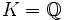# Artin L-function

Jump to: navigation, search
This term is related to: Galois theory
View other terms related to Galois theory | View facts related to Galois theory

This article defines a type of L-function

This article or section of article is sourced from:Wikipedia

## Definition

Let$L/K$ be a Galois extension of fields, and$G$ its Galois group. Let$\rho$be a linear representation of$G$ over$\mathbb{C}$. (In other words,$\rho$ is a Galois representation over the complex numbers).

The Artin L-function associated with$\rho$, denoted as$s \mapsto L(\rho,s)$, is defined as follows: it is the product, over all prime ideals$P$, of the following Euler factor corresponding to that$P$:$det(1 - t\rho(Frob(P)))^{-1}$

evaluated at$t = N(P^{-s})$.

Strictly speaking, the above definition works when$P$ is unramified. A slight variant works when$P$ is ramified.

PLACEHOLDER FOR INFORMATION TO BE FILLED IN: [SHOW MORE]

## Particular cases

### For Abelian Galois group

When the underlying Galois group$G$ is Abelian, the Artin L-function specializes to the Hecke L-function.

### For Abelian Galois group and over rationals

When$G$ is Abelian and$K = \mathbb{Q}$, the Artin L-function specializes to the Dirichlet L-function.